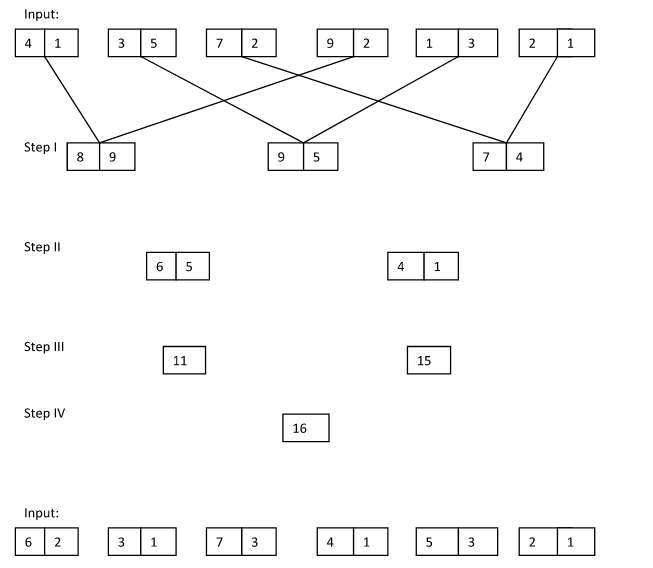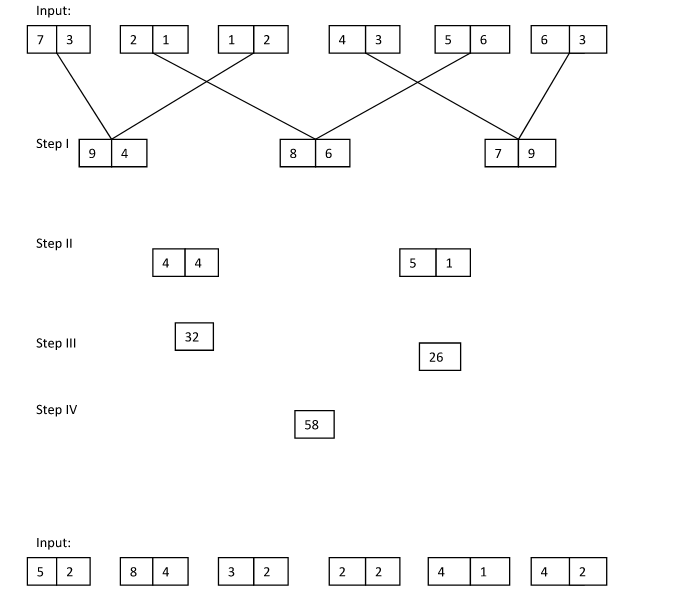# Crack IBPS Clerk Mains– Logical Reasoning (New Pattern Input Output)

## Crack IBPS Clerk Mains– Logical Reasoning (New Pattern Input Output):

Dear Readers, IBPS is conducting Online Examination for the recruitment of Clerical Cadre. Main Examination of  IBPS Clerk was scheduled from 21st Jan 2018. To enrich your preparation here we have providing new series of Practice Questions on Reasoning Ability -New Pattern Input Output.Candidates those who are appearing in IBPS Clerk Exam can practice these questions daily and make your preparation effective.

[WpProQuiz 1103]

### Click “Start Quiz” to attend these Questions and view Solutions

Directions (1-5): Study the following information carefully and answer the given questions.

A number arrangement machine arranges two digit numbers into a typical manner. Each step gives output taking input from the previous step. The following is an illustration of Input and rearrangement. Using the illustration answer the question given below.1. What is the multiplication of two numbers obtained in step III?
1. 253
2. 241
3. 236
4. 222
5. None of these
1. If each digit in the step II is add with value “2” and then added, then what will be the final sum?
1. 32
2. 48
3. 26
4. 18
5. None of these
1. Which of the following represent the difference between the first digit of the second number and second digit of the first number in step II?
1. 4
2. 5
3. 3
4. 2
5. None of these
1. If the value ‘25^2’ is subtracted from the final output, then what will be the resultant value?
1. 62
2. 51
3. 45
4. 33
5. None of these
1. Which of the following combination represent the first digit of the third number and second digit of the first number in step I of the given input?
1. 6, 9
2. 5, 8
3. 9, 7
4. 7, 8
5. None of these

Directions (6-10): Study the following information carefully and answer the givenquestions.

A number arrangement machine arranges two digit numbers into a typical manner. Each step gives output taking input from the previous step. The following is an illustration of Input and rearrangement. Using the illustration answer the question given below.1. Which of the following combination represent the first digit of the third number and second digit of the first number in step I of the given input?
1. 4, 5
2. 9, 7
3. 8, 6
4. 6, 4
5. None of these
1. If each digit in the step II is halved and then added, then what will be the final sum?
1. 8
2. 11
3. 12.5
4. 9.5
5. None of these
1. What is the difference of two numbers obtained in step III?
1. 29
2. 37
3. 41
4. 45
5. None of these
1. If the value ‘5^3’ is added with the final output, then what will be the resultant value?
1. 220
2. 335
3. 410
4. 195
5. None of these
1. Which of the following represent the difference between the first digit of the second number and second digit of the first number in step II?
1. 0
2. 1
3. 2
4. 4
5. None of these